# NCERT Solutions - Sound, Science, Class 9 Notes | Study Class 9 Science by VP Classes - Class 9

## Class 9: NCERT Solutions - Sound, Science, Class 9 Notes | Study Class 9 Science by VP Classes - Class 9

The document NCERT Solutions - Sound, Science, Class 9 Notes | Study Class 9 Science by VP Classes - Class 9 is a part of the Class 9 Course Class 9 Science by VP Classes.
All you need of Class 9 at this link: Class 9

Q1. How does the sound produced by a vibrating object in a medium reach your ear?
Ans.
When a disturbance is created in an object, it starts vibrating and sets the particles of the medium to vibrate. The particle of the medium is displaced from its mean position and exerts a force in the adjacent particle. As a result, the adjacent particle is disturbed from its mean position and the original particle comes back to rest. This process continues till the disturbance reaches our ear.

Q2. Explain how sound is produced by your school bell.
Ans
. When the bell is hit manually with a rod, the object, i.e. the bell starts vibrating causing disturbance in the medium, i.e. air. Here the air particles do not travel, they oscillate back and forth to transmit the disturbance till it reaches our ears.

Q3. Why are sound waves called mechanical waves?
Ans.
Mechanical waves are those which needs a medium for propagation. Sound waves are mechanical waves because they need a medium for propagation. If there is no medium, then sound cannot be propagated because it is the particle in the medium which oscillates back and forth and the disturbance is carried forward.

Q4. Suppose you and your friend are on the moon. Will you be able to hear any sound produced by your friend?
Ans.
No. We’ll not be able to hear the sound because sound requires a medium for its propagation. On the moon there is no atmosphere, i.e. there is vacuum. Sound can’t travel through vacuum, it needs a medium for its propagation.

Q5. Which wave property determines (a) loudness (b) pitch?
Ans.
(a) Loudness is determined by amplitude. If larger is the amplitude, louder will be the sound produced.
(b) Pitch is determined by its frequency. Higher the frequency, higher will be the pitch.

Q6. Guess which sound has a higher pitch: guitar or car horn?
Ans.
Car horn has the higher pitch.

Q7. What are wavelength, frequency, time period and amplitude of a sound wave?
Ans. Wavelength:
The distance between two consecutive compressions (Ω) or two consecutive rarefactions (R) is called the wavelength and its SI unit is metre.
Frequency: The number of oscillations per unit time is called frequency and its unit is Hertz (Hz).
Time period: The time taken by two consecutive compressions or rarefactions to cross a fixed point is called the time period.
Amplitude: The magnitude of the maximum disturbance in the medium on either side of the mean value is called the amplitude of the wave.

Q8. How are the wavelength and frequency of a sound wave related to its speed?
Ans.
The speed is defined as the distance travelled by a wave per unit time.

Speed = Distance / time

= λ/T

λ is the wavelength, T is the time period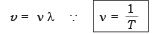∴ Speed = Wavelength × Frequency

Q9. Calculate the wavelength of a sound wave whose frequency is 220 Hz and speed is 440 m/s in a given medium.
Ans.
Speed = Wavelength × Frequency
ν = 220 Hz
v = 440 m/sec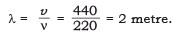Q10. A person is listening to a tone of 500 Hz sitting at a distance of 450 m from the source of the sound. What is the time interval between successive compressions from the source?
Ans
. ν = 500 Hz
λ = 450 m

v= 1/T

∴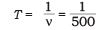= 0.002 second.

Q11. Distinguish between loudness and intensity of sound.
Ans.

 Intensity Loudness 1.The amount of sound energy passing each second per unit area. 1.It is the measure of response of the ear to the sound. 2.Intensity is a physical quantity and its magnitude does not depend on the senstivity of the ear. 2.Loudness depends upon the senstivity of ear.

Q12. In which of the three media, air, water or iron, does sound travel the fastest at a particular temperature?
Ans.
The speed of sound in air = 346 m/s.
The speed of sound in water = 1531 m/s.
The speed of sound in iron = 5950 m/s.
∴ The speed of sound in iron is greater and sound travels faster in iron.

Q13. An echo returned in 3 s. What is the distance of the reflecting surface from the source, given that the speed of sound is 342 ms–1?
Ans
. Speed of sound = 342 m/s.
Time taken for hearing an echo = 3 s.
Distance travelled 2d = v × t 2 × d = 342 × 3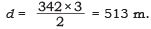Q14. Why are the ceilings of concert halls curved?
Ans.
Ceilings of the concert halls are curved because sound after reflection reaches all corners of the hall.

Q15. What is the audible range of the average human ear?
Ans.
The audible range of the average human ear is 20 Hz to 20000 Hz.

Q16. What is the range of frequencies associated with
(a) Infrasound?
(b) Ultrasound?
Ans.
(a) Sound of frequencies below 20 Hz are called infrasound.
(b) Sound of frequencies above 20 KHz are called ultrasound.

Q17. A submarine emits a sonar pulse which returns from an underwater cliff in 1.02 s. If the speed of sound in salt water is 1531 m/s, how far away is the cliff?
Ans
. Speed of sound in salt water = 1531 m/sec.
Time period = 1.02 sec.
Distance travelled 2 × d = speed × time
2 × d = 1531 × 1.02

∴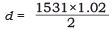d = 780.9 m.

NCERT Textbook Questions Solved

Q1. What is sound and how is it produced?
Ans.
Sound is a form of energy. It is produced when the object starts vibrating.

Q2. Describe with the help of a diagram, how compressions and rarefactions are produced in air near a source of sound.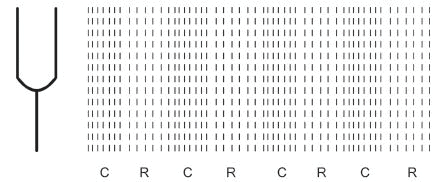Ans. (i) When a vibrating object moves forward, it pushes the air in front of it and compresses the air creating a region of high pressure called compression (C).
(ii) It starts moving away from the surface of the vibrating object.
(iii) As this occurs the surface moves backward creating a region of low pressure called rarefaction (R).

Q3. Cite an experiment to show that sound needs a material medium for its propagation.
Ans. Description:
Take an electric bell and an air tight glass bell jar. The electric bell is suspended inside an air tight glass jar which is connected to a vacuum pump.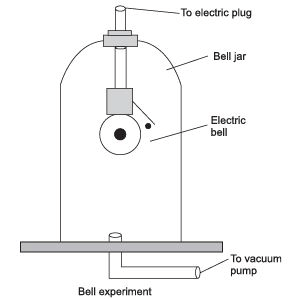Working: (i) When we press the switch, we’ll be able to hear the bell.
(ii) When the air in the jar is pumped out gradually, the sound becomes feeble although the same amount of current is flowing through the bell.
(iii) When the air is removed completely, we’ll not be able to hear the sound of the bell.
Conclusion: This experiment shows that sound requires a medium for its propagation.

Q4. Why is sound wave called a longitudinal wave?
Ans.
When the sound wave travels, the air molecules oscillate in the same direction as that of propagation of sound waves. Hence they are called longitudinal waves.

Q5. Which characteristic of the sound helps you to identify your friend by his voice while sitting with others in a dark room?
Ans.
The characteristic ‘Quality’ help us to identify our friend by his voice, although we are not actually seeing him.

Q6. Flash and thunder are produced simultaneously. But thunder is heard a few seconds after the flash is seen, why?
Ans
. Speed of sound is 330 m/sec in air medium at 0°C. Whereas speed of light is 3 × 10m/sec. When we compare the speed of light with that of speed of sound, speed of light is greater than that of speed of sound. Therefore thunder is heard a few seconds after the flash is seen.

Q7. A person has a hearing range from 20 Hz to 20 kHz. What are the typical wavelengths of sound waves in air corresponding to these two frequencies? Take the speed of sound in air as 344 ms–1.
Ans.
When ν = 20 Hz
Speed of sound in air = 344 m/s.
wavelength, λ = ?
Speed = Wavelength × Frequency

∴ Wavelength = Speed / Frequency = 344/20 = 17.2 m

when ν = 20 kHz

Speed of sound in air = 344 m/s.

λ = ?

(speed), v = ν × λ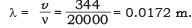Thus, the wavelength of sound corresponding to 20 Hz and 20 kHz is 17.2 m and 0.0172 m respectively.

Q8. Two children are at opposite ends of an aluminium rod. One strikes the end of the rod with a stone. Find the ratio of times taken by the sound wave in air and in aluminium to reach the second child.
Ans.
Speed of sound in air, V1 = 346 m/sec.
Speed of sound in aluminium, V= 6420 m/sec.
Let the length of the aluminium rod = x m.

We know that speed = distance / time

∴   Time = distance/ speed

Time taken in air = x/346 sec.  [∵ distance = x m]

time taken in aluminium = x/6420 sec.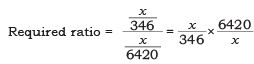Q9. The frequency of a source of sound is 100 Hz. How many times does it vibrate in a minute?
Ans
. Frequency of sound = 100 Hz.
Time taken = 1 minute = 60 sec We know,

Frequency =  No. of oscillations/ Time taken

No. of oscillations = ν × t = 100 × 60 = 6000 times.

Q10. Does sound follow the same laws of reflection as light does? Explain.
Ans.
Yes, Sound follows the same laws of reflection as that of light because
(i) Angle of incidence of sound is always equal to that of angle of reflection of sound waves.
(ii) The direction in which sound is incident, the direction in which it is reflected and normal all lie in the same plane.

Q11. When a sound is reflected from a distant object, an echo is produced. Let the distance between the reflecting surface and the source of sound production remains the same. Do you hear echo sound on a hotter day?
Ans.
Time = distance/ speed

Time is inversely proportional to the speed. As the temperature increases, the speed increases. Thus on a hot day due to high temperature the speed of sound increases. Hence the time will decrease and we can hear the echo sooner.

Q12. Give two practical applications of reflection of sound waves.
Ans.
Reflection of sound is used in megaphones, horns and musical instruments such as trumpets and shehnais. It is used in stethoscope for hearing patient’s heartbeat. Ceilings of the concert halls are curved, so that sound after reflection reaches all corners of the hall. (Any two practical applications can be written).

Q13. A stone dropped from the top of a tower 500 m high into a pond of water at the base of the tower. When is the splash heard at the top? Giving, g = 10 ms–2 and speed of sound = 340 ms–1.
Ans.
Distance covered by the stone = 500 m.
Initial velocity = 0 m/sec.
Acceleration due to gravity = 10 m/sec2.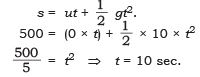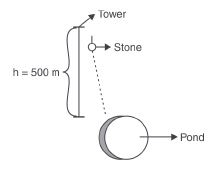Thus in 10 sec. the stone reaches the pond.
Speed of sound = 340 m/sec.
Time taken by sound to cover a distance of 500 m = ( = 1.47 sec
∴ Total time taken = 10 + 1.47 = 11.47 sec.
In 11.47 sec the splash will be heard on the top.

Q14. A sound wave travels at a speed of 339 ms–1. If its wavelength is 1.5 cm, what is the frequency of the wave? Will it be audible?
Ans.
Speed of sound = 339 m/sec.
Wavelength λ = 1.5 cm = 0.015 m.

Frequency =  speed/wavelength = 339/0.015 = 22600 Hz.

It will not be audible.

Q15. What is reverberation? How can it be reduced?
Ans.
The persistence of sound in an auditorium is the result of repeated reflections of sound and is called reverberation.
To reduce the undesirable effects due to reverberation, roofs and walls of the auditorium are generally covered with sound absorbent materials like compressed fiberboard, rough plaster or draperies. The seat materials are also selected having sound absorption properties.

Q16. What is loudness of sound? What factors does it depend on?
Ans.
The loudness of sound is determined by its amplitude. The amplitude of the sound wave depends upon the force with which an object is made to vibrate. Loud sound can travel a larger distance as it is associated with higher energy. A sound waves spreads out from its source. As it moves away from the source its amplitude as well as its loudness decreases.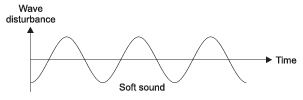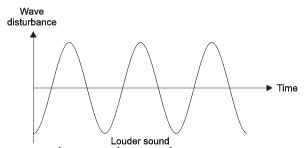Q17. Explain how bats use ultrasound to catch a prey.
Ans.
Bats search out its prey by emitting and detecting reflections of ultrasonic waves. The high-pitched ultrasonic squeaks of bat are reflected from the obstacles or prey and return to bat’s ear. The nature of reflection tells the bat where the obstacle or prey is and what it is like.

Q18. How is ultrasound used for cleaning?
Ans.
Ultrasound is used to clean parts located in hard-to-reach places (i.e.) spiral tube, odd shaped parts, electronic components etc. Objects to be cleaned are placed in a cleaning solution and ultrasonic waves are sent into the solution. Due to the high frequency, the dust particles, grease get detached and drop out. The objects thus get thoroughly cleaned.

Q19. Explain the working and application of a sonar.
Ans.
Working: SONAR consists of a transmitter and a detector and is installed in a boat or a ship as shown in the figure. The transmitter produces and transmits ultrasonic waves. These waves travel through water and after striking the object on the seabed, get reflected back and are sensed by the detector. The detector converts the ultrasonic waves into electrical signals which are appropriately interpreted. The distance of the object that reflected the sound wave can be calculated by knowing the speed of sound in water and the time interval between the transmission and reception of the ultrasound.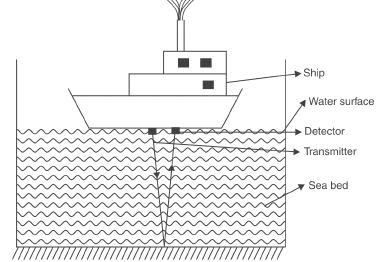Ultrasound sent by the transmitter and received by the detector
Let, Depth of the sea = d Speed of sound in sea water = v
Time taken for transmission and reception of signal = t
∴ Time taken to travel a distance, d =(t/2)
∴ Depth of the sea, d = (t/2) × v                                 (∵ distance = speed × time)

Q20. A sonar device on a submarine sends out a signal and receives an echo 5 s later. Calculate the speed of sound in water if the distance of the object from the submarine is 3625 m.
Ans
. Time taken between transmission and reception of signal = 5 sec.
Distance of the object from the submarine = 3625 m.
Distance travelled by the ultrasound = 3625 × 2 = 7250 m.
Speed of sound in water =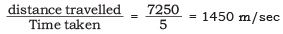Q21. Explain how defects in a metal block can be detected using ultrasound.
Ans.
Ultrasounds can be used to detect cracks and flaws in metal blocks. Metallic components are used in the construction of big structures like buildings, bridges, machines and scientific equipments. The cracks or holes inside the metal blocks, which are invisible from outside reduces the strength of the structure. Ultrasonic waves are allowed to pass through the metallic block and detectors are used to detect the transmitted waves. If there is even a small defect, the ultrasound gets reflected back indicating the presence of the flaw or defect.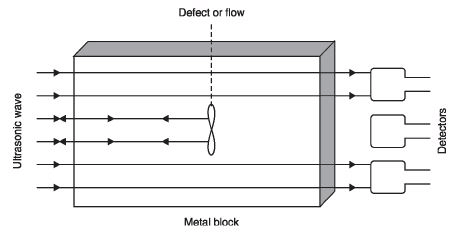Ultrasound    is    reflected    back    from    the    defective    locations    inside    a    metal    block

Q22. Explain how the human ear works.
Ans.
The outer ear is called ‘pinna. It collects the sound from the surroundings. The collected sound passes through the auditory canal. At the end of the auditory canal there is a thin membrane called the ear drum or the tympanic membrane. When a compression of the medium reaches the eardrum the pressure on the outside of the membrane increases and forces the eardrum inward. Similarly, the eardrum moves outward when a rarefaction reaches it. In this way the eardrum vibrates. The vibrations are amplified several times by three bones (the hammer, anvil and stirrup) in the middle ear. The middle ear transmits the amplified pressure variations received from the sound wave to the inner ear. In the inner ear, the pressure variations are turned into electrical signals by the cochlea. These electrical signals are sent to the brain via the auditory nerve and the brain interprets them as sound.

The document NCERT Solutions - Sound, Science, Class 9 Notes | Study Class 9 Science by VP Classes - Class 9 is a part of the Class 9 Course Class 9 Science by VP Classes.
All you need of Class 9 at this link: Class 9Use Code STAYHOME200 and get INR 200 additional OFF

## Class 9 Science by VP Classes

75 docs|14 tests

Track your progress, build streaks, highlight & save important lessons and more!

,

,

,

,

,

,

,

,

,

,

,

,

,

,

,

,

,

,

,

,

,

,

,

,

,

,

,

;